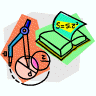## Mathematics, Department of## Faculty Publications, Department of Mathematics

9-21-2006

#### Citation

2006 Authors

Published as Norming Algebras and Automatic Complete Boundedness of Isomorphisms of Operator Algebras, Proceedings of the American Mathematical Society, 136(5), (2008), 1757-1768.

#### Abstract

We combine the notion of norming algebra introduced by Pop, Sinclair and Smith with a result of Pisier to show that if A1 and A2 are operator algebras, then any bounded epimorphism of A1 onto A2 is completely bounded provided that A2 contains a norming C*-subalgebra. We use this result to give some insights into Kadison’s Similarity Problem: we show that every faithful bounded homomorphism of a C*-algebra on a Hilbert space has completely bounded inverse, and show that a bounded representation of a C-algebra is similar to a -representation precisely when the image operator algebra -norms itself. We give two applications to isometric isomorphisms of certain operator algebras. The first is an extension of a result of Davidson and Power on isometric isomorphisms of CSL algebras. Secondly, we show that an isometric isomorphism between subalgebras Ai of C*-diagonals (Ci,Di) (i = 1, 2) satisfying Di Ai Ci extends uniquely to a - isomorphism of the C*-algebras generated by A1 and A2; this generalizes results of Muhly-Qiu-Solel and Donsig-Pitts.

COinS